Next: Example 8.2: Charged particle Up: Magnetism Previous: Worked Examples

## Example 8.1: Earth's magnetic field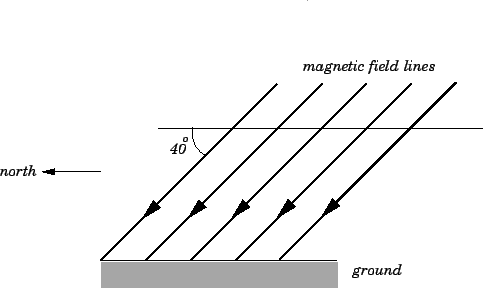Question: In Texas, the Earth's magnetic field is approximately uniform, and of magnitude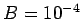T. The horizontal component of the field is directed northward. The field also has a vertical component which is directed into the ground. The angle the field lines dip below the horizontal is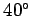. A metal bar of length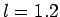m carries a current of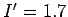A. Suppose that the bar is held horizontally such that the current flows from East to West. What is the magnitude and direction of the magnetic force on the bar? Suppose that the direction of the current is reversed. What, now, is the magnitude and direction of the magnetic force on the bar? Suppose that the bar is held vertically such the current flows upward. What is the magnitude and direction of the magnetic force on the bar? Suppose, finally, that the direction of the current is reversed. What, now, is the magnitude and direction of the magnetic force on the bar?

Answer: If the current in the bar flows horizontally from East to West then the direction of the current makes an angle of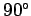with the direction of the magnetic field. So, from Eq. (152), the magnetic force per unit length acting on the bar is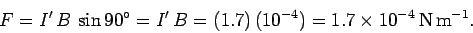Thus, the total force acting on the bar is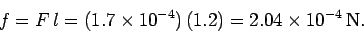Using the right-hand rule, if the index finger of a right-hand points horizontally from East to West, and the middle finger points northward, but dipsbelow the horizontal, then the thumb points southward, but dips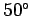below the horizontal. Thus, the force on the bar is directed southward, and dipsbelow the horizontal. If the current in the bar is reversed, so that it now flows horizontally from West to East, then the angle subtended between the direction of current flow and the direction of the magnetic field is still, so the magnitude of the force on the bar remains unchanged. According to the right-hand rule, if the index finger of a right-hand points horizontally from West to East, and the middle finger points northward, but dipsbelow the horizontal, then the thumb points northward, but is directedabove the horizontal. Thus, the force on the bar is directed northward at an angle ofabove the horizontal. In other words, the new force points in exactly the opposite direction to the old one.

If the current in the bar flows vertically upward then the direction of current flow subtends an angle ofdegrees with the direction of the magnetic field. So, from Eq. (152), the magnetic force per unit length acting on the bar is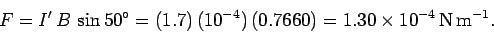Thus, the total force acting on the bar is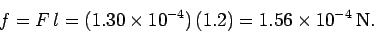Using the right-hand rule, if the index finger of a right-hand points vertically upward, and the middle finger points northward, but dipsbelow the horizontal, then the thumb points horizontally westward. Thus, the force on the bar is directed horizontally westward. If the current in the bar is reversed, so that it flows vertically downward, then the force on the bar is of the same magnitude, but points in the opposite direction, which means that the new force points horizontally eastward.Next: Example 8.2: Charged particle Up: Magnetism Previous: Worked Examples
Richard Fitzpatrick 2007-07-14